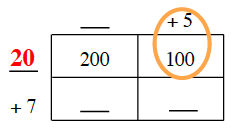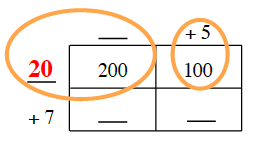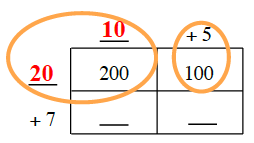Home > CC2MN > Chapter cc23 > Lesson cc23.1.1 > Problem3-11

3-11.

Copy and complete the generic rectangle below. What multiplication problem does it represent and what is the product? Homework Help ✎

 _____ $+5$ _____ $200$ $100$ $+7$ _____ _____

You know that $5(\text{something}) = 100$. What is that something?

You know that $20(\text{something}) = 200$. What is that something?

Complete the rest of the square using multiplication.